# Find the Impendance

#### arhzz1

Joined Oct 21, 2020
42
Determine the impedance Zx so that UAB is independent of any load
Z is. No double fractions may appear in the result.
By which component can the impedance Zx
will be realized? What value has
this component?
Note: The result Zx → ∞ is not sought.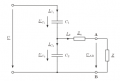I've used a method we were shown in class where we simply close the circuit on the left (meaning there will be wires where U is) and go from point A to point B.That would mean that these capacitors are in series. Now I've put calculated Zx like this;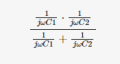Now if my algebra is not faulty we can make the denominator look like this;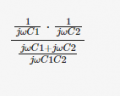After simplifying;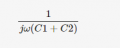Now the solution should be;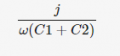I do not understand how they get to this.We've solved a similar problem like this in class and I'm pretty sure the method I used is not the problem but rather my algebra,or lack of if you will. Any insights?

Many thanks!

#### MrChips

Joined Oct 2, 2009
25,918
Can you see that

$$\frac{1}{j} = - j$$

#### arhzz1

Joined Oct 21, 2020
42
Can you see that

1 / j = - j
Yes,if I would to put j in the nummerator it would be negative,but I need it to be positive

#### MrChips

Joined Oct 2, 2009
25,918
The minus sign means that there is 180° phase difference.